Home | | Maths 6th Std | Types and Properties of Triangles

# Types and Properties of Triangles

If three sides of a triangle are different in lengths, then it is called a Scalene Triangle

Types and Properties of Triangles

Some triangles are drawn in the dotted sheet. Try to draw as many triangles as you can. Then, measure the sides and angles of all triangles and fill the table given below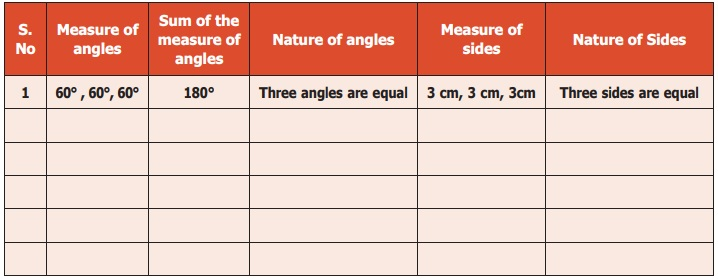From the table, we observe the following:

In a triangle,

* If the measure of all angles are different, then all sides are different.

* If the measure of two angles are equal, then two sides are equal.

* If the measure of three angles are equal, then three sides are equal and each angle measures 60°

Sum of three angles of a triangle is 180° .

Activity

Students are divided into groups and each group is given 3 sticks of length 9 units, 2 sticks of length 3 units, 2 sticks of length 2 units, 1 stick of length 5 units and 1 stick of length 4 units. Using the given sticks they are asked to form three triangles, find the length of the sides of each triangle and tabulate them.1. Was each group able to form 3 triangles?

2. In each of the triangle formed, how many sides are equal?

1. Types of triangle based on its sides

i) If three sides of a triangle are different in lengths, then it is called a Scalene Triangle

Examples:ii) If any two sides of a triangle are equal in length, then it is called an Isosceles Triangle

Examples: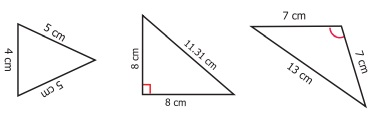iii) If three sides of a triangle are equal in length, then it is called an Equilateral Triangle

Examples: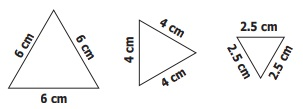Thus, based on the sides of triangles, we can classify triangles into 3 types.

Try these

Complete the following table. In any triangle,2. Types of triangle based on its angles

Write the given angles as acute, obtuse or right angle formed by two line segments AB and AC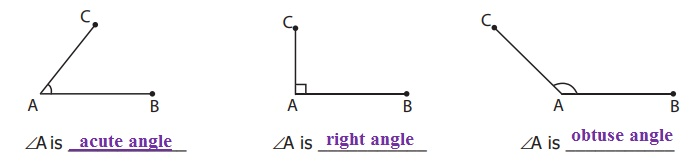Now, join the third side to form a triangle in each case and identify the kinds of angles and list them down.A is acute angle

B is acute angle

C is acute angleA is right angle

B is acute angle

C is acute angleA is obtuse angle

B is acute angle

C is acute angle

Now carefully look at these three triangles,

(i) If three angles of a triangle are acute angles (between 0° and 90°), then it is called an Acute Angled Triangle.

Examples: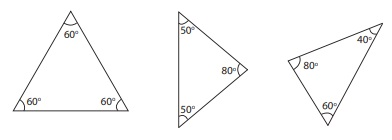ii) If an angle of a triangle is a right angle (90°), then it is called a Right Angled Triangle.

Examples: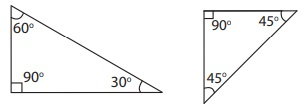iii) If an angle of a triangle is an obtuse angle (between 90° and 180° ), then it is called an Obtuse Angled Triangle.

Examples:Thus, based on the angles of triangles, we can classify triangles into 3 types.

Try these

Complete the tableDO YOU KNOW

A triangle can have three acute angles, but cannot have more than one right angle or an obtuse angle.Activity

In the given figure, there are some triangles. Measure their sides and angles and name them in two ways. (One is done for you )3. Triangle Inequality property

Three students Kamala, Madhan and Sumathi are asked to form triangles with the given sticks of measure 6cm, 8cm, 5cm; 4cm, 10cm, 5cm and 10cm, 6cm, 4cm respectively. All of them try to form a triangle. While Kamala, the first girl is successful in forming a triangle, Madhan and Sumathi, next to Kamala are struggling. Why?When they are trying to join the ends of the two smaller sticks, they find that the two smaller sticks coincide with the longer stick or shorter than the longer stick and they are unable to form triangles. From this, they understand that,

To form a triangle the sum of two smaller sides must be greater than the third side. Thus,

In a triangle, the sum of any two sides of a triangle is greater than the third side.

This is known as Triangle Inequality property.AB + BC > CA

BC + CA > AB

CA + AB > BC

Note

If three sides are equal in length, then definitely a triangle can be formed

DO YOU KNOW

If two sides of the triangle  are given, then the length of the third side will lie between the difference and sum of the lengths of two given sides.

Example 1:

Can a triangle be formed with 7 cm, 10 cm and 5 cm as its sides

Solution: Instead of checking triangle inequality by all the sides in the triangle, check only with two smaller sides.

Sum of two smaller sides of the triangle = 5+7=12 cm > 10 cm, the third side.

It is greater than the third side.

So, a triangle can be formed with the given sides.

Example 2:

Can a triangle be formed with 7 cm, 7 cm and 7 cm as its sides?

Solution:

If three sides are equal, then definitely a triangle can be formed, as the triangle inequality is satisfied.

Example 3:

Can a triangle be formed with 8 cm, 3 cm and 4 cm as its sides?

Solution:

The sum of two smaller sides = 3+4=7 cm < 8 cm, the third side.

It is less than the third side.

So, a triangle cannot be formed with the given sides.

Try these

Can a triangle be formed with the given sides? If yes, state the type of triangle formed.Example 4:

Can a triangle be formed with the angles 80°, 30° , 40°?

Solution:

The sum of three angles = 80° + 30° + 40° = 150° (not equal to 180° )

In a triangle, the sum of three angles is 180°.

So, a triangle cannot be formed with the given angles.

Think

Can the difference between two larger sides be less than the third side?

Activity

A triangle game: In each turn a student must draw one line connecting two dots. A line should not cross other lines or touch other dots than the two that are connected to. If a student closes a triangle with his line then he gets a point. Once there are no more lines that can be drawn the game is over and the student who gains more points wins the game.Think

In a right triangle, what measures can the other two angles have?

Tags : Geometry | Term 2 Chapter 4 | 6th Maths , 6th Maths : Term 2 Unit 4 : Geometry
Study Material, Lecturing Notes, Assignment, Reference, Wiki description explanation, brief detail
6th Maths : Term 2 Unit 4 : Geometry : Types and Properties of Triangles | Geometry | Term 2 Chapter 4 | 6th Maths